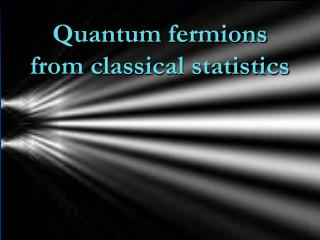DownloadDownload PresentationQuantum fermions from classical statistics

# Quantum fermions from classical statistics

Download Presentation## Quantum fermions from classical statistics

- - - - - - - - - - - - - - - - - - - - - - - - - - - E N D - - - - - - - - - - - - - - - - - - - - - - - - - - -
##### Presentation Transcript

1. Quantum fermionsfrom classical statistics

2. quantum mechanics can be described by classical statistics !

3. quantumparticle from classical probabilities

4. Double slit experiment Is there a classical probability density w(x,t) describing interference ? Suitable time evolution law : local , causal ? Yes ! Bell’s inequalities ? Kochen-Specker Theorem ? Or hidden parameters w(x,α,t) ? or w(x,p,t) ?

5. statistical picture of the world • basic theory is not deterministic • basic theory makes only statements about probabilities for sequences of events and establishes correlations • probabilism is fundamental , not determinism ! quantum mechanics from classical statistics : not a deterministic hidden variable theory

6. Probabilistic realism Physical theories and laws only describe probabilities

7. Gott würfelt Gott würfelt nicht Physics only describes probabilities

8. Physics only describes probabilities Gott würfelt

9. but nothing beyond classical statistics is needed for quantum mechanics !

10. fermions from classical statistics

11. Classical probabilities for two interfering Majoranaspinors Interference terms

12. Ising-type lattice model x : points on lattice n(x) =1 : particle present , n(x)=0 : particle absent

13. microphysical ensemble • states τ • labeled by sequences of occupation numbers or bits ns = 0 or 1 • τ = [ ns ] = [0,0,1,0,1,1,0,1,0,1,1,1,1,0,…] etc. • s=(x,γ) • probabilities pτ > 0

14. (infinitely) many degrees of freedom s = ( x , γ ) x : lattice points , γ : different species number of values of s : B number of states τ : 2^B

15. Classical wave function Classical wave function q is real , not necessarily positive Positivity of probabilities automatic.

16. Time evolution Rotation preserves normalization of probabilities Evolution equation specifies dynamics simple evolution : R independent of q

17. Grassmann formalism Formulation of evolution equation in terms of action of Grassmann functional integral Symmetries simple , e.g. Lorentz symmetry for relativistic particles Result : evolution of classical wave function describes dynamics of Dirac particles Dirac equation for wave function of single particle state Non-relativistic approximation : Schrödinger equation for particle in potential

18. Grassmann wave function Map between classical states and basis elements of Grassmann algebra s = ( x , γ ) For every ns= 0 : gτ contains factor ψs Grassmann wave function :

19. Functional integral Grassmann wave function depends on t , since classical wave function q depends on t ( fixed basis elements of Grassmann algebra ) Evolution equation for g(t) Functional integral

20. Wave function from functional integral L(t) depends only on ψ(t) and ψ(t+ε)

21. Evolution equation Evolution equation for classical wave function , and therefore also for classical probability distribution , is specified by action S Real Grassmann algebra needed , since classical wave function is real

22. MasslessMajoranaspinors in four dimensions

23. Time evolution linear in q , non-linear in p

24. One particle states : arbitrary static “vacuum” state One –particle wave function obeys Dirac equation

25. Dirac spinor in electromagnetic field one particle state obeys Dirac equation complex Dirac equation in electromagnetic field

26. Schrödinger equation Non – relativistic approximation : Time-evolution of particle in a potential described by standard Schrödinger equation. Time evolution of probabilities in classical statistical Ising-type model generates all quantum features of particle in a potential , as interference ( double slit ) or tunneling. This holds if initial distribution corresponds to one-particle state.

27. what is an atom ? • quantum mechanics : isolated object • quantum field theory : excitation of complicated vacuum • classical statistics : sub-system of ensemble with infinitely many degrees of freedom

28. i

29. Phases and complex structure introduce complex spinors : complex wave function :

30. h

31. Simple conversion factor for units

32. [A,B]=C

33. non-commuting observables classical statistical systems admit many product structures of observables many different definitions of correlation functions possible , not only classical correlation ! type of measurement determines correct selection of correlation function ! example 1 : euclidean lattice gauge theories example 2 : function observables

34. function observables

35. microphysical ensemble • states τ • labeled by sequences of occupation numbers or bits ns = 0 or 1 • τ = [ ns ] = [0,0,1,0,1,1,0,1,0,1,1,1,1,0,…] etc. • probabilities pτ > 0

36. function observable

37. function observable normalized difference between occupied and empty bits in interval s I(x1) I(x2) I(x3) I(x4)

38. generalized function observable normalization classical expectation value several species α

39. position classical observable : fixed value for every state τ

40. momentum • derivative observable classical observable : fixed value for every state τ

41. complex structure

42. different products of observables differs from classical product

43. Which product describes correlations of measurements ?

44. coarse graining of informationfor subsystems

45. density matrix from coarse graining • position and momentum observables use only • small part of the information contained in pτ, • relevant part can be described by density matrix • subsystem described only by information • which is contained in density matrix • coarse graining of information

46. quantum density matrix density matrix has the properties of a quantum density matrix